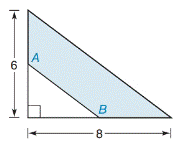Chapter 8.1, Problem 22E### Elementary Geometry for College St...

6th Edition
Daniel C. Alexander + 1 other
ISBN: 9781285195698

#### Solutions

Chapter
Section### Elementary Geometry for College St...

6th Edition
Daniel C. Alexander + 1 other
ISBN: 9781285195698
Textbook Problem
4 views

# In Exercises 19 to 22, find the area of the shaded region.A and B are midpoints.

To determine

To find:

The area of the shaded region.

Explanation

Finding the area of the figure is followed by identifying the figure and find area with the suitable geometry formulae.

Calculation:

Given,

This figure represents the right triangle inscribed in the larger right triangle A and B are midpoints.

Thus,

The area of the shaded region A=(A1) area of the larger right triangle(A2)area of the smaler right triangle (i.e.) A=A1A2

Area of the right triangle =12ab

Where, a and b the legs of a right triangle

Here, a1=6

a2=62 [since A and B are midpoints]

b1=8

### Still sussing out bartleby?

Check out a sample textbook solution.

See a sample solution

#### The Solution to Your Study Problems

Bartleby provides explanations to thousands of textbook problems written by our experts, many with advanced degrees!

Get Started

#### Find more solutions based on key concepts

Elementary Technical Mathematics

#### Expand each expression in Exercises 122. (x+1)(x3)

Finite Mathematics and Applied Calculus (MindTap Course List)

#### Convert from radians to degrees. 9. 512

Single Variable Calculus: Early Transcendentals, Volume I

#### Evaluate the iterated integral: 0202cosrdrd

Calculus: Early Transcendental Functions (MindTap Course List)

#### The scalar projection of on is:

Study Guide for Stewart's Multivariable Calculus, 8th